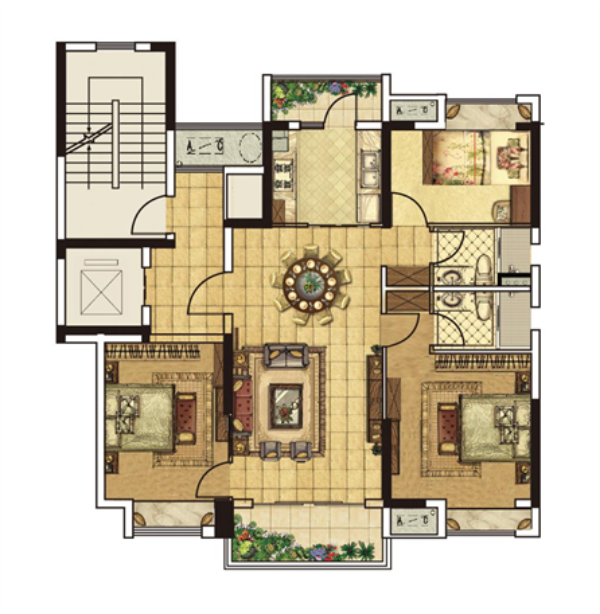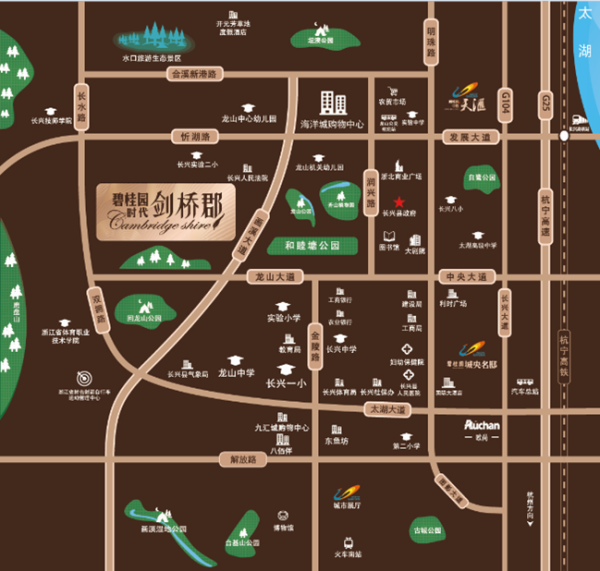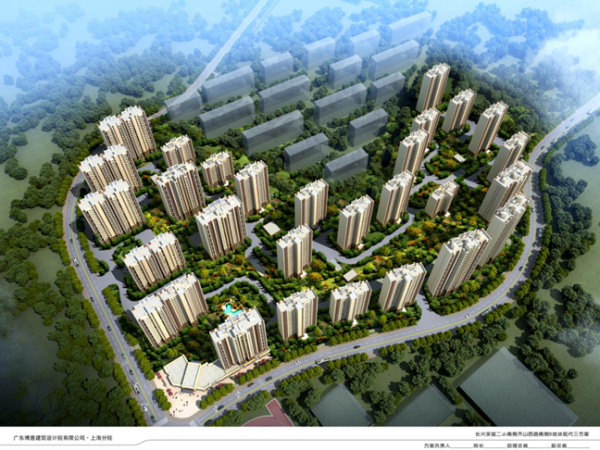## 网站地图## 长兴碧桂园时代•剑桥郡

### 湖州

#### 地址

• 资阳
• 本溪
• 北流
• 万载
• 慈溪
• 长春
• 通化
• 西昌
• 丰城
• 阳春
• 鹤山
• 江油
• 辛集
• 辽源
• 衡水
• 黔西南州
• 黔东南州
• 石家庄
• 唐山
• 秦皇岛
• 邯郸
• 邢台
• 保定
• 张家口
• 承德
• 沧州
• 廊坊
• 黄骅
• 迁安
• 怀来
• 洛阳
• 驻马店
• 郑州
• 开封
• 平顶山
• 安阳
• 鹤壁
• 新乡
• 焦作
• 濮阳
• 许昌
• 漯河
• 三门峡
• 南阳
• 商丘
• 信阳
• 周口
• 武汉
• 黄石
• 十堰
• 宜昌
• 襄阳
• 荆州
• 荆门
• 鄂州
• 仙桃
• 潜江
• 黄冈
• 随州
• 咸宁
• 恩施
• 怀化
• 长沙
• 株洲
• 湘潭
• 湘西州
• 张家界
• 益阳
• 郴州
• 衡阳
• 岳阳
• 常德
• 娄底
• 徐州
• 南京
• 无锡
• 镇江
• 泰州
• 淮安
• 舟山
• 黔西南布依族苗族自治州
• 临沂
• 凉山
• 普洱
• 红河
• 锦州
• 葫芦岛
• 东方
• 咸阳
• 丽水
• 定安县
• 吕梁
• 来宾
• 河池
• 宜宾
• 重庆市
• 雅安
• 潮州
• 大理
• 巴中
• 桂林
• 贵港
• 百色
• 柳州
• 贺州
• 儋州
• 福清
• 威海
• 烟台
• 乐山
• 金华
• 永泰
• 潍坊
• 滨州
• 德州
• 临汾
• 日照
• 眉山
• 武威
• 乌鲁木齐
• 保山
• 临沧
• 防城港
• 达州
• 遂宁
• 绵阳
• 淮南
• 宝鸡
• 枣庄
• 桂平
• 景德镇
• 临夏
• 聊城
• 崇左
• 马鞍山
• 安顺
• 北海
• 菏泽
• 铜陵
• 黔南州
• 蚌埠
• 嘉兴
• 淮北
• 都匀
• 南宁
• 兴义
• 南平
• 松溪
• 吉安
• 大同
• 陆丰
• 厦门
• 铜仁
• 银川
• 昭通
• 包头
• 遵化
• 漳州
• 深圳
• 霸州
• 香港
• 合肥
• 抚州
• 琼海
• 定西
• 南昌
• 宿州
• 文昌
• 临高县
• 陵水
• 海口
• 博鳌
• 三亚
• 绥化
• 哈尔滨
• 杭州
• 绍兴
• 宁波
• 温州
• 衢州
• 湖州
• 台州
• 天津
• 沈阳
• 大连
• 通辽
• 兴安盟
• 呼伦贝尔
• 运城
• 晋中
• 太原
• 南通
• 宿迁
• 扬州
• 盐城
• 连云港
• 苏州
• 常州
• 安庆
• 黄山
• 巢湖
• 芜湖
• 池州
• 宣城
• 六安
• 古田
• 无为
• 桐城
• 亳州
• 南陵
• 庐江
• 阜阳
• 滁州
• 孝感
• 天门
• 邵阳
• 永州
• 平果
• 玉林
• 梧州
• 钦州
• 梅州
• 河源
• 云浮
• 东莞
• 汕头
• 中山
• 清远
• 惠州
• 韶关
• 肇庆
• 阳江
• 汕尾
• 茂名
• 江门
• 佛山
• 广州
• 雷州
• 英德
• 湛江
• 揭阳
• 珠海
• 信宜
• 济南
• 淄博
• 青岛
• 泰安
• 济宁
• 马来西亚雪兰莪州
• 马来西亚柔佛州
• 永春
• 南安
• 三明
• 宁德
• 武夷山
• 福鼎
• 莆田
• 建瓯
• 漳浦
• 福安
• 福州
• 泉州
• 龙岩
• 兰州
• 张掖
• 天水
• 白银
• 贵阳
• 仁怀
• 毕节
• 凯里
• 六盘水
• 遵义
• 广元
• 德阳
• 南充
• 泸州
• 攀枝花
• 广安
• 成都
• 宜春
• 萍乡
• 九江
• 赣州
• 上饶
• 曲靖
• 楚雄
• 昆明
• 白城
• 北京
• 澳洲莱德
• 西安
• 渭南
• 上海
• 西宁

核心优势：

No1：龙山新区之中 城市发展动脉

龙山新区拥有县行政中心、大剧院、人民医院、体育馆等标志性建筑，集行政文化、商务、会展和居住功能为一体，是本县未来发展规划的重要区域。

No2：两纵两横一高铁 优越交通布局

两横分别是龙山大道、发展大道，两纵分别是画溪大道、长水路，一高铁为长兴高铁站，贯穿市区各个角落，通过高速及高铁可以到达沪宁杭等一线城市。

No3：三大商圈配套 畅想一站购物

1公里内有18万㎡海洋城购物中心，2公里到达浙北大厦、3公里到达利时广场等综合性购物中心，出则繁华、入则宁静。

No4：优质学府林立 教育资源优渥

剑桥郡距离长兴一小约2.2公里，距离龙山中学约1.4公里。另外，在项目北侧约50米的地方，就是长兴县第二实验小学。咫尺便是名校，教育资源优渥。

No5：五大公园环绕 舒适天然氧吧

新区环境优美、景色宜人，齐山植物公园、梅花公园、花菇山公园等五大公园环绕，天然氧吧，依托自然美景，茶余饭后一家人散步悠闲，享受健康的生活理念。

No6：上市物业公司 尊崇管家服务

碧桂园物业，2018中国物业服务三强，迄今为止已为超百万业主提供24小时360度的贴心管家式服务。

No7： 世界五百强 中国地产三强

中国地产三强碧桂园集团，连续两年荣登《财富》世界500强。

No8：加纳康生活配套 增添生活乐趣

项目配置游泳池、儿童游乐场、彩虹跑道、健身平台、按摩步道等健康设施，增添家人之间的互动，丰富业主的娱乐生活，成为茶余饭后的新去处。

No9：智慧社区规划 保障居住安全

碧桂园4.0智慧社区配备智能可视对讲、电子脉冲围栏、智能车辆识别等各项智能服务系统。接轨新科技，提供未来智能生活方式，让您开启智慧新生活。

No10：资深设计团队 精雕立体园林

碧桂园特邀中国资深设计大师，利用高尔夫球场设计经验，处理高低落差，避免使用挡土墙，实现“虽由人作，宛自天开”的效果。每一处都细心打造，真正做到目之所及，皆为美好。

户型：YJ115

三开间朝南，南向大尺度开间，阔绰视野，点亮生活

南北通透，客餐厅南北通透，通风采光性佳

南北双阳台，北向独立家政阳台，南向独立大面宽景观阳台YJ140

四开间朝南，南向大尺度开间，阔绰视野，点亮生活

南北通透，客餐厅南北通透，通风采光性佳

多飘窗设计，提高空间利用率YJ190

四开间朝南，南向大尺度开间，阔绰视野，点亮生活

南北通透，客餐厅南北通透，通风采光性佳

行政级主卧套房，私享空间、衣帽间、私属卫浴，媲美星级酒店豪华套房

双厨设置，两进室中西厨房，西厨兼顾早餐厅，更添生活品味YJ260

四开间朝南，南向大尺度开间，阔绰视野，点亮生活

南北通透，客餐厅南北通透，通风采光性佳

多飘窗设计，提高空间利用率

双套房式卧室，主次卧均配享独立衣帽间、卫生间，私密尊崇加倍

鸟瞰图+区位图+透视图：